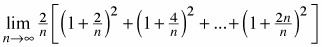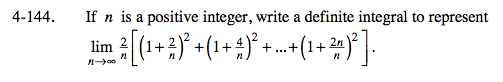### Home > CALC > Chapter 4 > Lesson 4.4.2 > Problem4-144

4-144.
1. If n is a positive integer, write a definite integral to represent. Homework Help ✎You should recognize this Riemann sum as the Definition of an Integral.

Thus, your job is to connect the given Riemann sum to the parts of an integral:

Start by answering these questions: What represents dx? What represents the integrand, f(x)? What are the bounds?

What represents dx?
dx is the infinitely small widths of the infinitely many rectangles.
dx can be represented by the expression

Substitute:

You probably want to factor out the 2.

What represents the integrand, f(x)?
In order to identify the f(x), you need to find the variable, x.
Look for the part of the series that is changing: the numerators!
The first numerator is 2, then 4, then 6, then ... 2n.
Now each variable is being divided by n .

because we are evaluating values x that are infinitely close together.
So f(x) = (1 + 2x)2
Substitute:

What are the bounds?
The bounds are the smallest input and the largest input into the variable.
Since the integrand is f(x) = (1 + 2x)2, where

Let's look at the smallest and largest value of x.
The smallest value of x is

$\frac{1}{n}, \text{ and }\lim_{n\rightarrow \infty }\frac{1}{n}=0$

The largest value of x is

So the bounds are form 0 to 1.
Substitute: